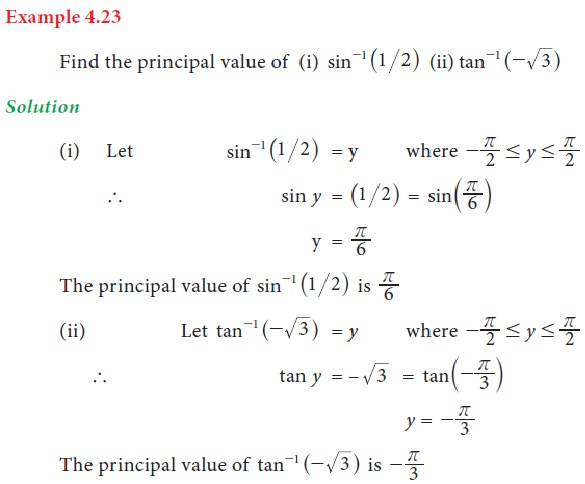Home | | Business Maths 11th std | Inverse Trigonometric Functions

# Inverse Trigonometric Functions

Business Mathematics and Statistics(EMS) : Trigonometry

Inverse Trigonometric Functions

## 1. Inverse Trigonometric Functions

The quantities such as sin-1 x , cos-1 x, tan-1 x etc., are known as inverse trigonometric functions.

i.e., if sin θ = x , then θ = sin-1 x . The domains and ranges (Principal value branches) of trigonometric functions are given below## 2. Properties of Inverse Trigonometric FunctionsTags : Definition, Formula, Solved Example Problems, Exercise | Mathematics , 11th Business Mathematics and Statistics(EMS) : Chapter 4 : Trigonometry
Study Material, Lecturing Notes, Assignment, Reference, Wiki description explanation, brief detail
11th Business Mathematics and Statistics(EMS) : Chapter 4 : Trigonometry : Inverse Trigonometric Functions | Definition, Formula, Solved Example Problems, Exercise | Mathematics